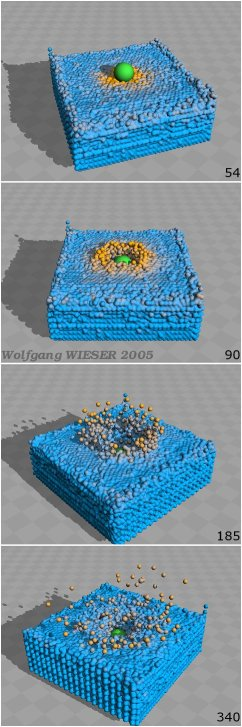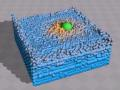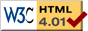Nav: [home] > [sim] > [ballimpact]

## Simple low-speed impact MD simulation

This is a simple 3d molecular dynamics (MD) simulation of a low-speed impact. This is a simple demo-code with rigid (spherical) body interaction including rotation and a simple phenomenological friction model with an easy-to-understand first-order ODE integrator (Euler method).

### What is being done

This is a molecular dynamics (MD) simulation of a number of spherical particles which are each characterized by radius, mass, inertia, position, velocity and angular velocity. The simulation computes the interaction forces of each particle with all other particles and numerically integrates the Newtonian equations of motion. I.e. the sum force on each particle gives its acceleration which is used to calculate new speed and position taking a tiny time step. With updated state (speed,...), the force is calculated again, etc.

The following forces are considered:

• Gravity force: Acts downwards.
• Force between particles: When particles touch, they exhibit a repulsive force on each other.
• Normal friction: Dissipation when particles collide (phenomenological model).
• Tangential friction: Dissipation when particles scrap along each other; this is a very simple model.

The simulation includes translational and angular velocities and also computes the corresponding forces, i.e. if two particles collide non-centrally, they spin afterwards.

The numerical integrator is an Euler-type first order integrator as explained above: With tiny time steps, forces give new (translational and angular) speed and new speed gives new position. Nothing fancy but very easy to understand.

And what can you do with it? For example the simulation of a low-speed impact in granular media as presented below. However, the number of balls in the sim is limited by your computer's performance...

### The simulation

The simulation program can be downloaded below. But please note that the source code presented here is not complete. The reason is that it depends on some external numerics templates which in turn depend on tons of other code. So, for now, I decided to only publish the simulation core while general math routines (such as cross product,...) are not included. Hence, if you want to play with it, you first need to understand what is being done to fill the gaps.

 Source: ballimpact.tar.gz   [7kb gzipped source tarball] Author: Wolfgang Wieser   (report bugs here) License: GNU GPL (Version 2) Requires: C++ (GCC >=3.4), QTXlib, Qt,...

When started, the simulation will compute the motion of all particles except the impact bullet ball. Leave the sim running this way for a while until the sand balls have settled down. Then, start the bullet ball by pressing 's'. This will also start the frame ouput.

The graphical display is an x-z projection of the 3d area: Looking into the screen you look along the y axis onto the x-z-plane. Only the center part (usually including the bullet ball) is displayed to keep things well visible. Fancy 3d visualisation can be done afterwards.

### Example resultsI took this simulation to the summer school of science (S3) where I led a project on impact craters in 2005 (see real low-speed impact photos). The students Quinn Burke and Slaven Glumac found the set of simulation parameters used in the images on the left; please refer to the source code for the meaning of these parameters:

```
const fp const_dt=0.00001/2;
const fp const_g=100;
const fp3 bullet_v0(6*0,0,-8);
const fp sand_ball_density=3000;
const fp bullet_ball_density=4000;
const int z_layers=20;
const fp stretch_fact=1.08*1.1;
const fp coeff_k = 1e6;
const fp delta_0 = 0.1*sand_ball_rad;
const fp coeff_nu=1.5;
const fp coeff_gamma_n=0.2*10;
const fp coeff_phi=1;
const fp coeff_gamma_t=0.1*10;
const fp coeff_mu=0.4;```

In the images on the left, color encodes translational velocity with a non-linear gradient from blue (slow) to yellow (fast).

Non-perpendicular impact film

This is an 17 second film showing a non-perpendicular impact; the parameters are the same as above except the initial bullet speed:

`const fp3 bullet_v0(6*1,0,-8);`

This time, color represents particle energy (blue to yellow).

The codec is MS MPEG4v2 (MP42) encoded using MPlayer's MEncoder. It can be viewed under Linux (I recommend MPlayer) as well as Windows.Download and view film: AVI 480x360, 1.2MBit/s (MS MPEG4v2) [2.7Mb]

(Balls strangely bouncing back in the film collide with the walls making up the "sand container". These walls were removed for the visualisation but are of course in the sim.)

### References

• Modeling granular media on the computer, H. J. Herrmann and S. Luding, Continuum Mech. Thermodyn. (1998) 10: 189-231
• How to simulate Billards and Similar Systems, Boris D. Lubachevsky, arXiv:cond-mat/0503627v1 (2005)